# PHP 协程实现

## 多进程/线程

``````\$serv = stream_socket_server("tcp://0.0.0.0:8000", \$errno, \$errstr)
or die("Create server failed");
while(1) {
\$conn = stream_socket_accept(\$serv);
if (pcntl_fork() == 0) {
// do something
// \$response = "hello world";
fwrite(\$response);
fclose(\$conn);
exit(0);
}
}复制代码``````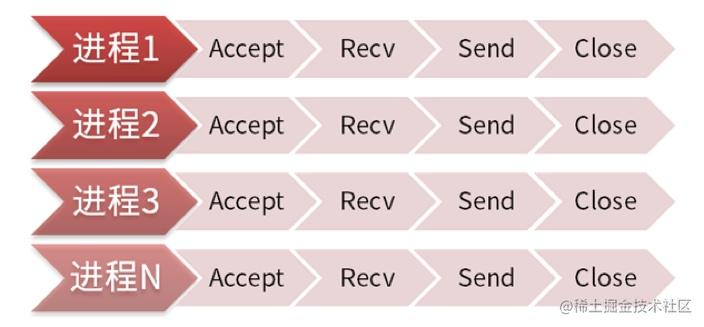``````\$serv = stream_socket_server("tcp://0.0.0.0:8000", \$errno, \$errstr)
or die("Create server failed");
for(\$i = 0; \$i < 32; \$i++) {
if (pcntl_fork() == 0) {
while(1) {
\$conn = stream_socket_accept(\$serv);
if (\$conn == false) continue;
// do something
// \$response = "hello world";
fwrite(\$response);
fclose(\$conn);
}
exit(0);
}
}复制代码``````

## 并行和并发

### 区别

• 『并发』指的是程序的结构，『并行』指的是程序运行时的状态
• 『并行』一定是并发的，『并行』是『并发』设计的一种
• 单线程永远无法达到『并行』状态

two tasks can start, run, and complete in overlapping time periods

## 迭代器 & 生成器

### 迭代器

PHP5 开始内置了 `Iterator` 即迭代器接口，所以如果你定义了一个类，并实现了`Iterator` 接口，那么你的这个类对象就是 `ZEND_ITER_OBJECT` 即可迭代的，否则就是 `ZEND_ITER_PLAIN_OBJECT`

#### Iterator 接口

``````interface Iterator extends Traversable
{
// 获取当前内部标量指向的元素的数据
public mixed current()
// 获取当前标量
public scalar key()
// 移动到下一个标量
public void next()
// 重置标量
public void rewind()
// 检查当前标量是否有效
public boolean valid()
}复制代码``````

#### 常规实现 range 函数

PHP 自带的 range 函数原型：

range — 根据范围创建数组，包含指定的元素

array range (mixed \$start , mixed \$end [, number \$step = 1 ])

``````function range (\$start, \$end, \$step = 1)
{
\$ret = [];

for (\$i = \$start; \$i <= \$end; \$i += \$step) {
\$ret[] = \$i;
}

return \$ret;
}复制代码``````

#### 迭代器实现 xrange 函数

``````class Xrange implements Iterator
{
protected \$start;
protected \$limit;
protected \$step;
protected \$current;
public function __construct(\$start, \$limit, \$step = 1)
{
\$this->start = \$start;
\$this->limit = \$limit;
\$this->step  = \$step;
}
public function rewind()
{
\$this->current = \$this->start;
}
public function next()
{
\$this->current += \$this->step;
}
public function current()
{
return \$this->current;
}
public function key()
{
return \$this->current + 1;
}
public function valid()
{
return \$this->current <= \$this->limit;
}
}复制代码``````

``````foreach (new Xrange(0, 9) as \$key => \$val) {
echo \$key, ' ', \$val, "\n";
}复制代码``````

``````0 0
1 1
2 2
3 3
4 4
5 5
6 6
7 7
8 8
9 9复制代码``````

``var_dump(new Xrange(0, 9));复制代码``

``````object(Xrange)#1 (4) {
["start":protected]=>
int(0)
["limit":protected]=>
int(9)
["step":protected]=>
int(1)
["current":protected]=>
NULL
}复制代码``````

``````// range
\$startMemory = memory_get_usage();
\$arr = range(0, 500000);
echo 'range(): ', memory_get_usage() - \$startMemory, " bytes\n";
unset(\$arr);
// xrange
\$startMemory = memory_get_usage();
\$arr = new Xrange(0, 500000);
echo 'xrange(): ', memory_get_usage() - \$startMemory, " bytes\n";复制代码``````

``````xrange(): 624 bytes
range(): 72194784 bytes复制代码``````

`range()` 函数在执行后占用了 50W 个元素内存空间，而 `xrange` 对象在整个迭代过程中只占用一个对象的内存。

#### Yii2 Query

``````\$query = (new \yii\db\Query)->from('user');
// yii\db\BatchQueryResult
foreach (\$query->batch() as \$users) {
// 每次循环得到多条 user 记录
}复制代码``````

``````/**
* Starts a batch query.
*
* A batch query supports fetching data in batches, which can keep the memory usage under a limit.
* This method will return a [[BatchQueryResult]] object which implements the [[\Iterator]] interface
* and can be traversed to retrieve the data in batches.
*
* For example,
*
*
* \$query = (new Query)->from('user');
* foreach (\$query->batch() as \$rows) {
*     // \$rows is an array of 10 or fewer rows from user table
* }
*
*
* @param integer \$batchSize the number of records to be fetched in each batch.
* @param Connection \$db the database connection. If not set, the "db" application component will be used.
* @return BatchQueryResult the batch query result. It implements the [[\Iterator]] interface
* and can be traversed to retrieve the data in batches.
*/
public function batch(\$batchSize = 100, \$db = null)
{
return Yii::createObject([
'class' => BatchQueryResult::className(),
'query' => \$this,
'batchSize' => \$batchSize,
'db' => \$db,
'each' => false,
]);
}复制代码``````

``````class BatchQueryResult extends Object implements \Iterator
{
public \$db;
public \$query;
public \$batchSize = 100;
public \$each = false;
private \$_batch;
private \$_value;
private \$_key;
/**
* Destructor.
*/
public function __destruct()
{
// make sure cursor is closed
\$this->reset();
}
/**
* Resets the batch query.
* This method will clean up the existing batch query so that a new batch query can be performed.
*/
public function reset()
{
}
\$this->_batch = null;
\$this->_value = null;
\$this->_key = null;
}
/**
* Resets the iterator to the initial state.
* This method is required by the interface [[\Iterator]].
*/
public function rewind()
{
\$this->reset();
\$this->next();
}
/**
* Moves the internal pointer to the next dataset.
* This method is required by the interface [[\Iterator]].
*/
public function next()
{
if (\$this->_batch === null || !\$this->each || \$this->each && next(\$this->_batch) === false) {
\$this->_batch = \$this->fetchData();
reset(\$this->_batch);
}
if (\$this->each) {
\$this->_value = current(\$this->_batch);
if (\$this->query->indexBy !== null) {
\$this->_key = key(\$this->_batch);
} elseif (key(\$this->_batch) !== null) {
\$this->_key++;
} else {
\$this->_key = null;
}
} else {
\$this->_value = \$this->_batch;
\$this->_key = \$this->_key === null ? 0 : \$this->_key + 1;
}
}
/**
* Fetches the next batch of data.
* @return array the data fetched
*/
protected function fetchData()
{
// ...
}
/**
* Returns the index of the current dataset.
* This method is required by the interface [[\Iterator]].
* @return integer the index of the current row.
*/
public function key()
{
return \$this->_key;
}
/**
* Returns the current dataset.
* This method is required by the interface [[\Iterator]].
* @return mixed the current dataset.
*/
public function current()
{
return \$this->_value;
}
/**
* Returns whether there is a valid dataset at the current position.
* This method is required by the interface [[\Iterator]].
* @return boolean whether there is a valid dataset at the current position.
*/
public function valid()
{
return !empty(\$this->_batch);
}
}复制代码``````

#### 迭代器使用场景

• 使用返回迭代器的包或库时（如 PHP5 中的 SPL 迭代器）
• 无法在一次调用获取所需的所有元素时
• 要处理数量巨大的元素时（数据库中要处理的结果集内容超过内存）

### 生成器

#### 生成器实现 `xrange` 函数

``````function xrange(\$start, \$limit, \$step = 1) {
for (\$i = 0; \$i < \$limit; \$i += \$step) {
yield \$i + 1 => \$i;
}
}
foreach (xrange(0, 9) as \$key => \$val) {
printf("%d %d \n", \$key, \$val);
}
// 输出
// 1 0
// 2 1
// 3 2
// 4 3
// 5 4
// 6 5
// 7 6
// 8 7
// 9 8复制代码``````

#### yield 关键字

`yield` 最简单的调用形式看起来像一个 `return` 申明，不同的是 `yield` 暂停当前过程的执行并返回值，而 `return` 是中断当前过程并返回值。暂停当前过程，意味着将处理权转交由上一级继续进行，直到上一级再次调用被暂停的过程，该过程又会从上一次暂停的位置继续执行。这像是什么呢？如果之前已经在鸟哥的文章中粗略看过，应该知道这很像操作系统的进程调度，多个进程在一个 CPU 核心上执行，在系统调度下每一个进程执行一段指令就被暂停，切换到下一个进程，这样外部用户看起来就像是同时在执行多个任务。

``````function printer()
{
while (true) {
}
}
\$printer = printer();
\$printer->send('hello');
\$printer->send('world');
// 输出

``````function printer()
{
\$i = 0;
while (true) {
}
}
\$printer = printer();
printf("%d\n", \$printer->current());
\$printer->send('hello');
printf("%d\n", \$printer->current());
\$printer->send('world');
printf("%d\n", \$printer->current());
// 输出
1
2
3复制代码``````

``````function gen() {
\$ret = (yield 'yield1');
var_dump(\$ret);
\$ret = (yield 'yield2');
var_dump(\$ret);
}

\$gen = gen();
var_dump(\$gen->current());    // string(6) "yield1"
var_dump(\$gen->send('ret1')); // string(4) "ret1"   (第一个 var_dump)
// string(6) "yield2" (继续执行到第二个 yield，吐出了返回值)
var_dump(\$gen->send('ret2')); // string(4) "ret2"   (第二个 var_dump)
// NULL (var_dump 之后没有其他语句，所以这次 ->send() 的返回值为 null)复制代码``````

`current` 方法是迭代器 `Iterator` 接口必要的方法，`foreach` 语句每一次迭代都会通过其获取当前值，而后调用迭代器的 `next` 方法。在上述例子里则是手动调用了 `current` 方法获取值。

• 对于所有在生成器函数中出现的 `yield`，首先它都是语句，而跟在 `yield` 后面的任何表达式的值将作为调用生成器函数的返回值，如果 `yield` 后面没有任何表达式（变量、常量都是表达式），那么它会返回 `NULL`，这一点和 `return` 语句一致。
• `yield` 也是表达式，它的值就是 `send` 函数传过来的值（相当于一个特殊变量，只不过赋值是通过 `send` 函数进行的）。只要调用send方法，并且生成器对象的迭代并未终结，那么当前位置的 `yield` 就会得到 `send` 方法传递过来的值，这和生成器函数有没有把这个值赋值给某个变量没有任何关系。

• `Next()`，恢复生成器函数的执行直到下一个 `yield`
• `Send()`，向生成器传入一个值，恢复执行直到下一个 `yield`

## 协程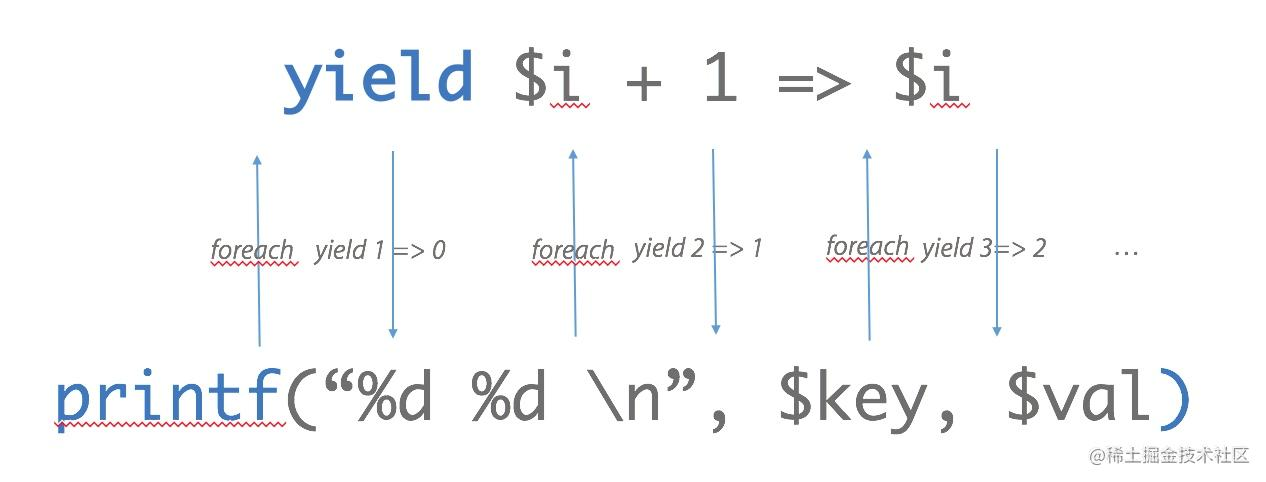### PHP 协程和 yield

PHP 从 5.5 开始支持生成器及 `yield` 关键字，而 PHP 协程则由 `yield` 来实现。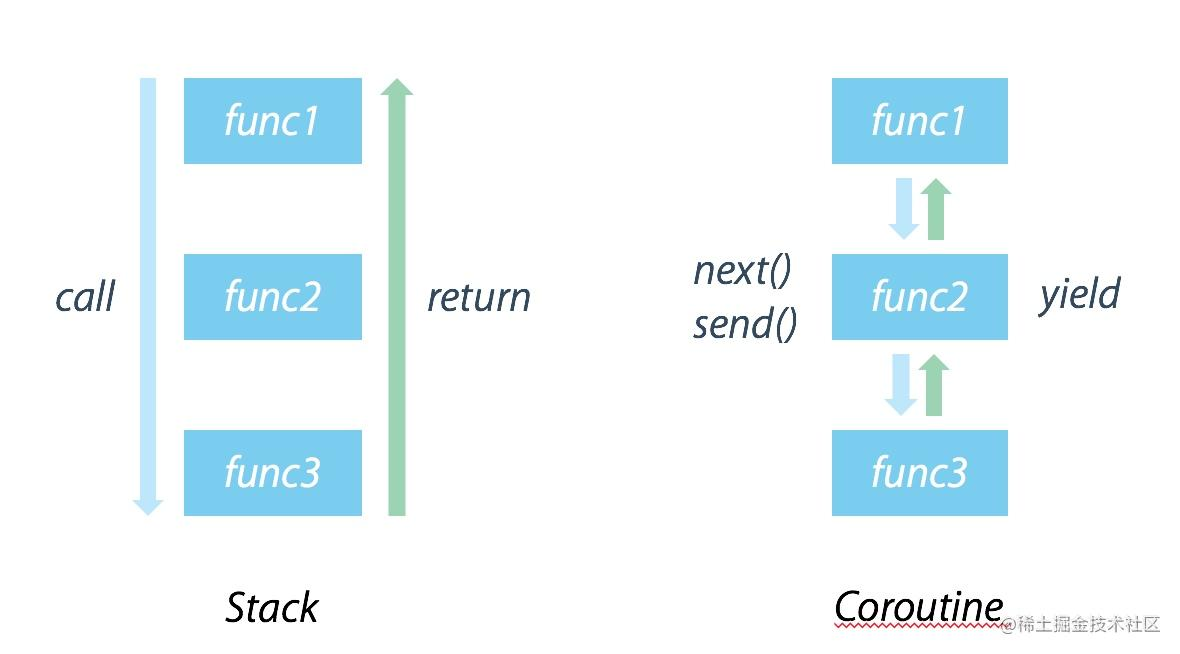• 实现不同任务间的主动让位、让行，把控制权交回给任务调度器。
• 通过 `send()` 实现不同任务间的双向通信，也就可以实现任务和调度器之间的通信。

`yield` 就是 PHP 实现协程的方式。

### 协程多任务调度

``````class Task
{
// 任务 ID
// 协程对象
protected \$coroutine;
// send() 值
protected \$sendVal = null;
// 是否首次 yield
protected \$beforeFirstYield = true;
public function __construct(\$taskId, Generator \$coroutine) {
\$this->coroutine = \$coroutine;
}

}
public function setSendValue(\$sendVal) {
\$this->sendVal = \$sendVal;
}
public function run() {
// 如之前提到的在send之前, 当迭代器被创建后第一次 yield 之前，一个 renwind() 方法会被隐式调用
// 所以实际上发生的应该类似:
// \$this->coroutine->rewind();
// \$this->coroutine->send();

// 这样 renwind 的执行将会导致第一个 yield 被执行, 并且忽略了他的返回值.
// 真正当我们调用 yield 的时候, 我们得到的是第二个yield的值，导致第一个yield的值被忽略。
// 所以这个加上一个是否第一次 yield 的判断来避免这个问题
if (\$this->beforeFirstYield) {
\$this->beforeFirstYield = false;
return \$this->coroutine->current();
} else {
\$retval = \$this->coroutine->send(\$this->sendVal);
\$this->sendVal = null;
return \$retval;
}
}
public function isFinished() {
return !\$this->coroutine->valid();
}
}复制代码``````

Scheduler

``````class Scheduler
{

public function __construct() {
}

// （使用下一个空闲的任务id）创建一个新任务,然后把这个任务放入任务map数组里. 接着它通过把任务放入任务队列里来实现对任务的调度. 接着run()方法扫描任务队列, 运行任务.如果一个任务结束了, 那么它将从队列里删除, 否则它将在队列的末尾再次被调度。
return \$tid;
}

// 任务入队
}

public function run() {
while (!\$this->queue->isEmpty()) {
// 任务出队

} else {
}
}
}
}复制代码``````

Demo

``````function task1() {
for (\$i = 1; \$i <= 10; ++\$i) {
echo "This is task 1 iteration \$i.\n";
yield;
}
}

for (\$i = 1; \$i <= 5; ++\$i) {
echo "This is task 2 iteration \$i.\n";
yield;
}
}

\$scheduler = new Scheduler;

\$scheduler->run();复制代码``````

``````This is task 1 iteration 1.
This is task 2 iteration 1.
This is task 1 iteration 2.
This is task 2 iteration 2.
This is task 1 iteration 3.
This is task 2 iteration 3.
This is task 1 iteration 4.
This is task 2 iteration 4.
This is task 1 iteration 5.
This is task 2 iteration 5.
This is task 1 iteration 6.
This is task 1 iteration 7.
This is task 1 iteration 8.
This is task 1 iteration 9.
This is task 1 iteration 10.复制代码``````

### 协程非阻塞 IO

``````<?php
class Scheduler
{
protected \$queue;
protected \$waitingForWrite = [];

public function __construct() {
// SPL 队列
\$this->queue = new SplQueue();
}

return \$tid;
}

// 任务入队
}

public function run() {
while (!\$this->queue->isEmpty()) {
// 任务出队

} else {
}
}
}
{
} else {
}
}
{
if (isset(\$this->waitingForWrite[(int)\$socket])) {
} else {
}
}
/**
* @param \$timeout 0 represent
*/
protected function ioPoll(\$timeout)
{
\$rSocks = [];
\$rSocks[] = \$socket;
}
\$wSocks = [];
foreach (\$this->waitingForWrite as list(\$socket)) {
\$wSocks[] = \$socket;
}
\$eSocks = [];
// \$timeout 为 0 时, stream_select 为立即返回，为 null 时则会阻塞的等，见 http://php.net/manual/zh/function.stream-select.php
if (!@stream_select(\$rSocks, \$wSocks, \$eSocks, \$timeout)) {
return;
}
foreach (\$rSocks as \$socket) {
}
}
foreach (\$wSocks as \$socket) {
unset(\$this->waitingForWrite[(int)\$socket]);
}
}
}
/**
* 检查队列是否为空，若为空则挂起的执行 stream_select，否则检查完 IO 状态立即返回，详见 ioPoll()
* 作为任务加入队列后，由于 while true，会被一直重复的加入任务队列，实现每次任务前检查 IO 状态
* @return Generator object for newTask
*
*/
{
while (true) {
\$this->ioPoll(null);
} else {
\$this->ioPoll(0);
}
yield;
}
}
/**
* \$scheduler = new Scheduler;
* \$scheduler->withIoPoll()->run();
*
* 新建 Web Server 任务后先执行 withIoPoll() 将 ioPollTask() 作为任务入队
*
* @return \$this
*/
public function withIoPoll()
{
return \$this;
}
}复制代码``````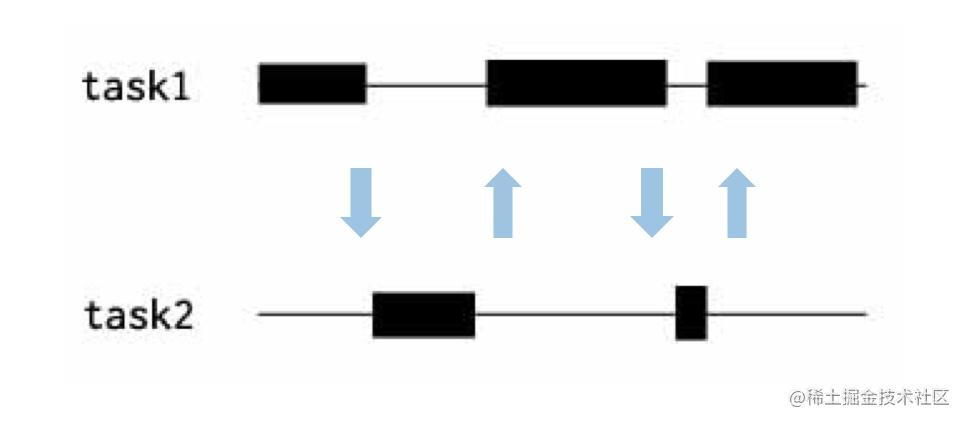### 单任务改造

• 多进程，不改变任务执行的整体过程，在一个时间段内同时执行多个相同的代码段，调度权在 CPU，如果一个任务能独占一个 CPU 则可以实现并行。
• 协程，把原有任务拆分成多个小任务，原有任务的执行流程被改变，调度权在进程自己，如果有 IO 并且可以实现异步，则可以实现并行。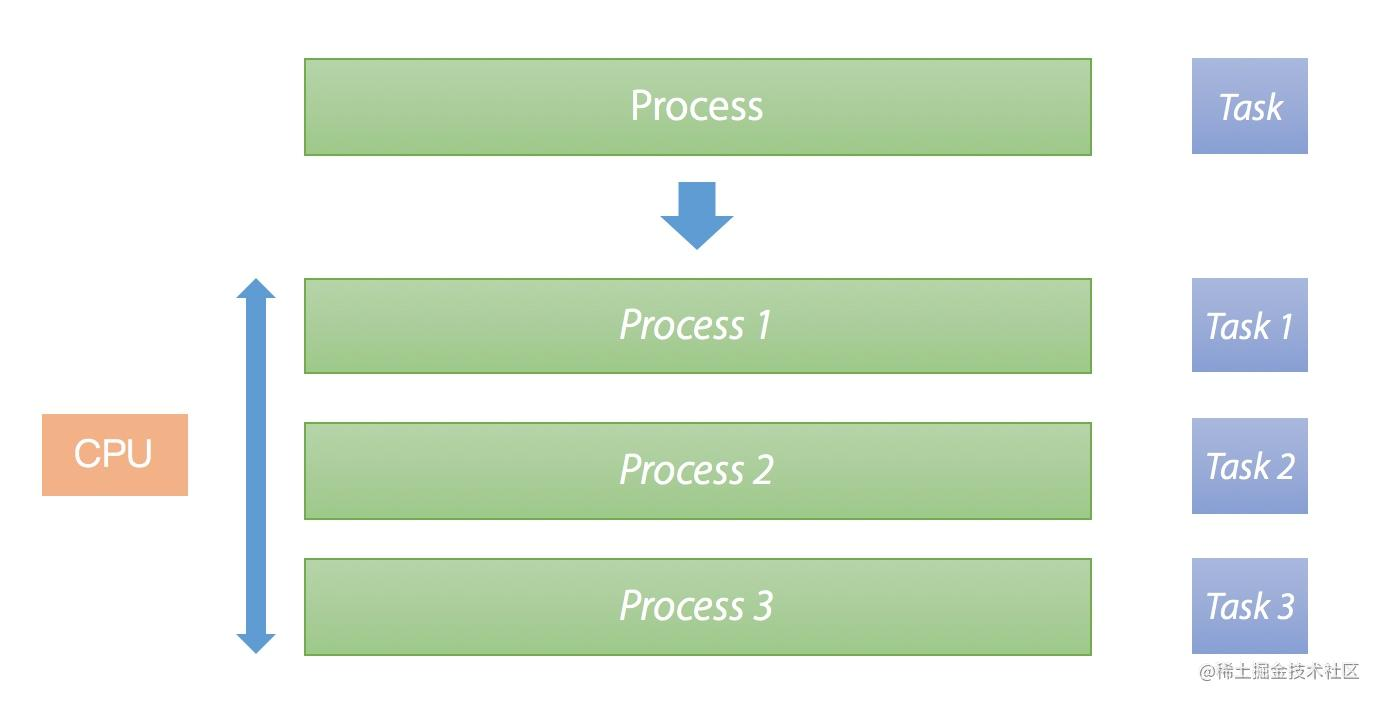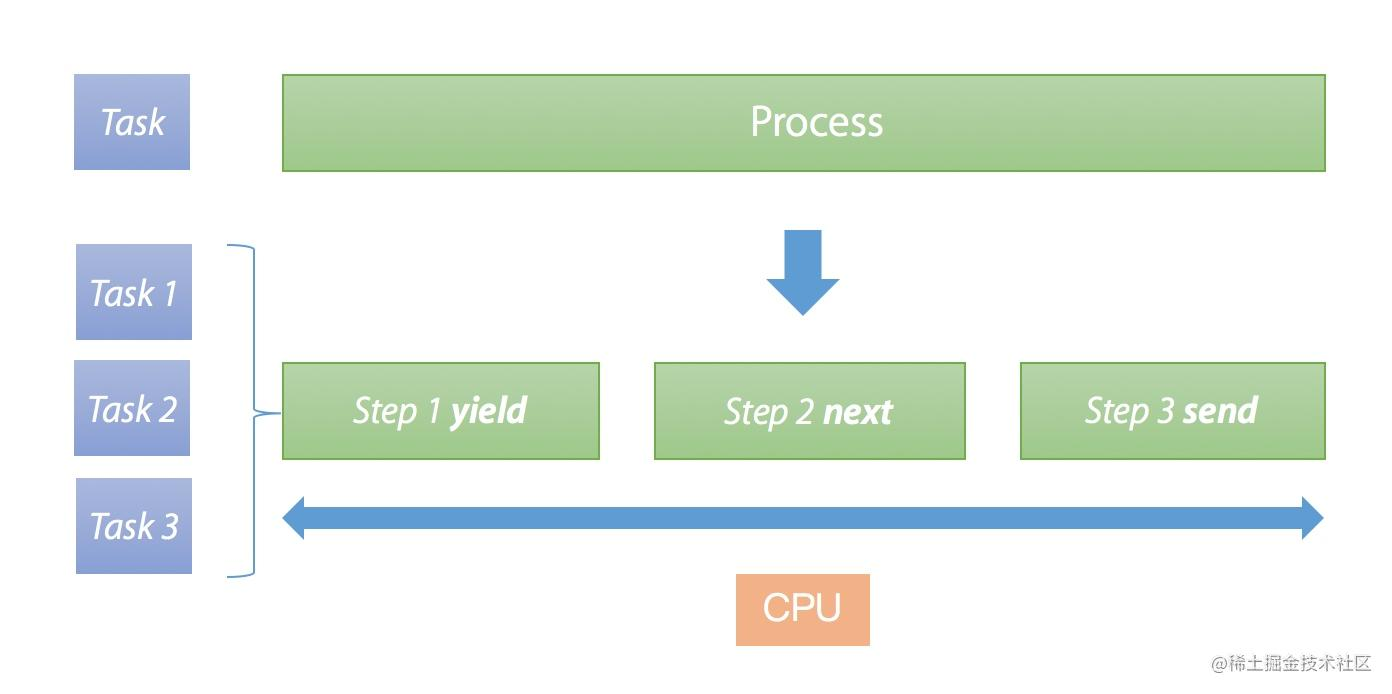## 协程（Coroutines）和 Go 协程（Goroutines）

PHP 的协程或者其他语言中，比如 Python、Lua 等都有协程的概念，和 Go 协程有些相似，不过有两点不同：

• Go 协程意味着并行（或者可以以并行的方式部署，可以用 `runtime.GOMAXPROCS()` 指定可同时使用的 CPU 个数），协程一般来说只是并发。
• Go 协程通过通道 `channel` 来通信；协程通过 `yield` 让出和恢复操作来通信。

Go 协程比普通协程更强大，也很容易从协程的逻辑复用到 Go 协程，而且在 Go 的开发中也使用的极为普遍，有兴趣的话可以了解一下作为对比。